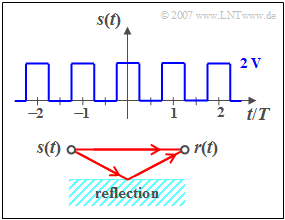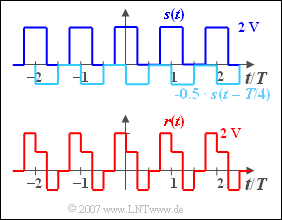# Exercise 3.7Z: Rectangular Signal with EchoTransmission signal  $s(t)$, and
reception signal  $r(t)$  with echo

We consider a periodic rectangular signal  $s(t)$  with the possible amplitude values  $0\text{ V}$  and  $2\text{ V}$  and the period duration  $T_0 = T = 1 \text{ ms}$.  At the jump points, e.g. at  $t = T/4$, the signal value are  $1\text{ V}$.  The DC component  $($i.e. the Fourier coefficient  $A_0)$  of the signal is  $1\text{ V}$, too.

Further applies:

• Due to symmetry (even function), all sine coefficients  $B_n = 0$.
• The coefficients  $A_n$  with even  $n$  are also zero.
• For odd values of  $n$,  the following applies:
$$A_n = ( { - 1} )^{\left( {n - 1} \right)/2} \cdot \frac{{4\;{\rm{V}}}}{{n \cdot {\rm{\pi }}}}.$$

The signal  $s(t)$  reaches the receiver via two paths (see sketch below):

• Once on the direct path and secondly via a secondary path.
• The latter is characterised by the attenuation factor  $\alpha$  and the transit time  $\tau$ .
• Therefore, the following applies to the received signal:
$$r(t) = s(t) + \alpha \cdot s( {t - \tau } ).$$

The frequency response of the channel is  $H(f) = R(f)/S(f)$,  the impulse response is denoted by  $h(t)$ .

Hints:

### Questions

1

Which statements are true regarding the impulse response  $h(t)$?

 For  $0 ≤ t < \tau$  holds  $h(t) = 1$, and for  $t > \tau$  holds   $h(t) = 1 + \alpha$. It holds that  $h(t) = \delta (t) + \alpha \cdot \delta(t - \tau)$. $h(t)$  has a Gaussian shape.

2

Calculate the reception signal  $r(t)$  for the channel parameters  $\alpha = -0.5$  and  $\tau = T/4$.
What values result at the given times?

 $r(t = 0.2 \cdot T)\ = \$  $\text{V}$ $r(t = 0.3 \cdot T)\ = \$  $\text{V}$

3

Calculate the reception signal  $r(t)$  with  $\alpha = 1$  and  $\tau = T/2$.  Interpret the result in the frequency domain.
What value results for  $t = T/2$?

 $r(t = T/2)\ = \$  $\text{V}$

### Solution

#### Solution

(1)  The second suggested solution is correct:

• The impulse response is equal to the received signal  $r(t)$, if a single Dirac delta is present at the input at time  $t = 0$ :
$$h(t) = \delta (t) + \alpha \cdot \delta( {t - \tau } ).$$Convolution of square wave signal  $s(t)$  and impulse response  $h(t)$

(2)  It holds  $r(t) = s(t) ∗ h(t)$.  This convolution operation is most easily performed graphically:

The values of the received signal are generally:

• $0.00 < t/T < 0.25\text{:}\hspace{0.4cm} r(t) = +1\hspace{0.02cm}\text{ V}$,
• $0.25 < t/T < 0.50\text{:}\hspace{0.4cm} r(t) = -1 \hspace{0.02cm}\text{ V}$,
• $0.50 < t/T < 0.75\text{:}\hspace{0.4cm} r(t) = 0 \hspace{0.02cm}\text{ V}$,
• $0.75 < t/T < 1.00\text{:}\hspace{0.4cm} r(t) = +2 \hspace{0.02cm}\text{ V}$.

The values we are looking for are thus

$$r(t = 0.2 \cdot T) \hspace{0.15cm}\underline{= +1 \hspace{0.02cm}\text{ V}},$$
$$r(t = 0.3 · T) \hspace{0.15cm}\underline{= -1 \hspace{0.02cm}\text{ V}}.$$

(3)  Using a similar procedure as in  (2),  a direct signal  $\rm (DC)$  of  $2\hspace{0.02cm}\text{ V}$  is obtained for  $r(t)$ :

• The gaps in the signal  $s(t)$  are completely filled by the echo  $s(t - T/2)$.
• This result can also be derived in the frequency domain.  The channel frequency response is with  $\alpha = 1$  and  $\tau = T/2$:
$$H( f ) = 1 + 1 \cdot {\rm{e}}^{ - {\rm{j\pi }}fT} = 1 + \cos ( {{\rm{\pi }}fT} ) - {\rm{j}} \cdot {\rm{sin}}( {{\rm{\pi }}fT} ).$$
• Apart from the DC component, the input signal  ${s(t)}$  only has components at  $f = f_0 = 1/T$,  $f = 3 \cdot f_0$,  $f = 5 \cdot f_0$,  etc..
• At these frequencies, however, both the real– and the imaginary part of  ${H(f)}$  are equal to zero.
• Thus, for the output spectrum with  $A_0 = 1 \text{ V}$  and  $H(f = 0) = 2$  we obtain:
$$R(f) = A_0 \cdot H(f = 0) \cdot \delta (f) = 2\;{\rm{V}} \cdot \delta (f).$$

The inverse Fourier transformation thus also yields  $r(t) \underline{= 2 \text{ V= const}}$.